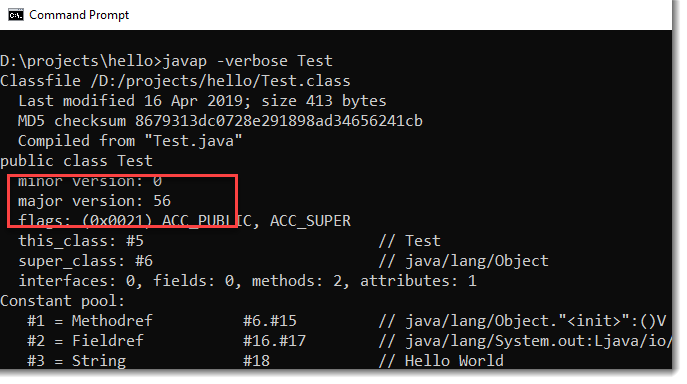How to check which JDK version compiled the class?In Java, we can use javap -verbose className to print out the class information.

Terminal

D:\projects>javap -verbose Test

Classfile /D:/projects/Test.class
Last modified 16 Apr 2019; size 413 bytes
Compiled from "Test.java"
public class Test
minor version: 0
major version: 56
flags: (0x0021) ACC_PUBLIC, ACC_SUPER
this_class: #5                          // Test
super_class: #6                         // java/lang/Object
interfaces: 0, fields: 0, methods: 2, attributes: 1
//...

Find the major version and match the following table :

Java SE 13 = 57 (0x39 hex)
Java SE 12 = 56 (0x38 hex)
Java SE 11 = 55 (0x37 hex)
Java SE 10 = 54 (0x36 hex)
Java SE 9 = 53 (0x35 hex)
Java SE 8 = 52 (0x34 hex)
Java SE 7 = 51 (0x33 hex)
Java SE 6.0 = 50 (0x32 hex)
Java SE 5.0 = 49 (0x31 hex)
JDK 1.4 = 48 (0x30 hex)
JDK 1.3 = 47 (0x2F hex)
JDK 1.2 = 46 (0x2E hex)
JDK 1.1 = 45 (0x2D hex)

Source : Wikipedia

For major version: 56, this Java class is compiled with Java SE 12

References